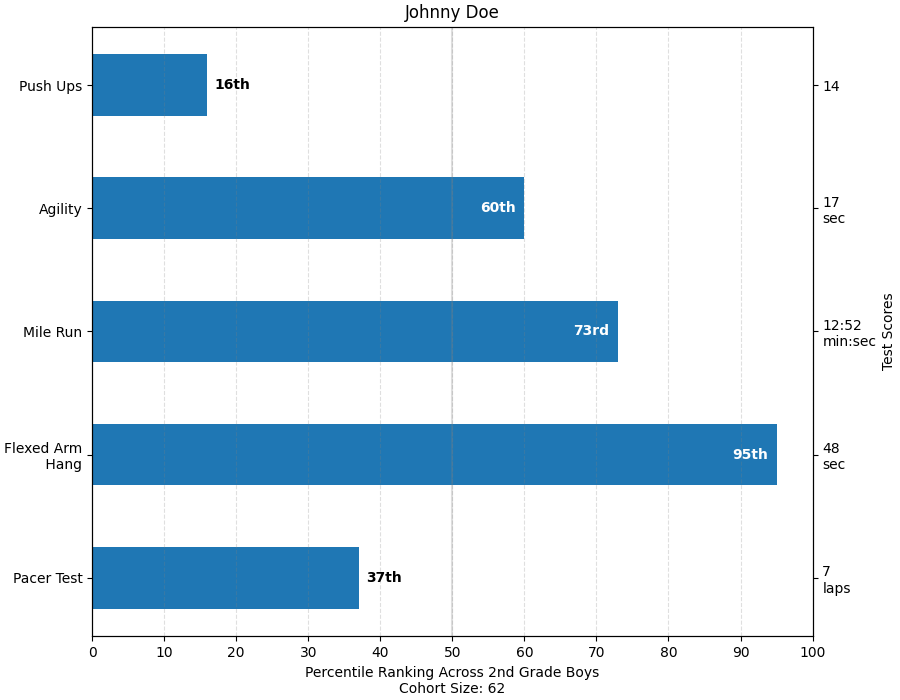# Percentiles as horizontal bar chart#

Bar charts are useful for visualizing counts, or summary statistics with error bars. Also see the Grouped bar chart with labels or the Horizontal bar chart example for simpler versions of those features.

This example comes from an application in which grade school gym teachers wanted to be able to show parents how their child did across a handful of fitness tests, and importantly, relative to how other children did. To extract the plotting code for demo purposes, we'll just make up some data for little Johnny Doe.

from collections import namedtuple
import numpy as np
import matplotlib.pyplot as plt

Student = namedtuple('Student', ['name', 'grade', 'gender'])
Score = namedtuple('Score', ['value', 'unit', 'percentile'])

def to_ordinal(num):
"""Convert an integer to an ordinal string, e.g. 2 -> '2nd'."""
suffixes = {str(i): v
for i, v in enumerate(['th', 'st', 'nd', 'rd', 'th',
'th', 'th', 'th', 'th', 'th'])}
v = str(num)
# special case early teens
if v in {'11', '12', '13'}:
return v + 'th'
return v + suffixes[v[-1]]

def format_score(score):
"""
Create score labels for the right y-axis as the test name followed by the
measurement unit (if any), split over two lines.
"""
return f'{score.value}\n{score.unit}' if score.unit else str(score.value)

def plot_student_results(student, scores_by_test, cohort_size):
fig, ax1 = plt.subplots(figsize=(9, 7), constrained_layout=True)

ax1.set_title(student.name)
ax1.set_xlabel(
'Cohort Size: {cohort_size}'.format(
gender=student.gender.title(),
cohort_size=cohort_size))

test_names = list(scores_by_test.keys())
percentiles = [score.percentile for score in scores_by_test.values()]

rects = ax1.barh(test_names, percentiles, align='center', height=0.5)
# Partition the percentile values to be able to draw large numbers in
# white within the bar, and small numbers in black outside the bar.
large_percentiles = [to_ordinal(p) if p > 40 else '' for p in percentiles]
small_percentiles = [to_ordinal(p) if p <= 40 else '' for p in percentiles]
ax1.bar_label(rects, small_percentiles,
ax1.bar_label(rects, large_percentiles,

ax1.set_xlim([0, 100])
ax1.set_xticks([0, 10, 20, 30, 40, 50, 60, 70, 80, 90, 100])
ax1.xaxis.grid(True, linestyle='--', which='major',
color='grey', alpha=.25)
ax1.axvline(50, color='grey', alpha=0.25)  # median position

# Set the right-hand Y-axis ticks and labels
ax2 = ax1.twinx()
# Set equal limits on both yaxis so that the ticks line up
ax2.set_ylim(ax1.get_ylim())
# Set the tick locations and labels
ax2.set_yticks(
np.arange(len(scores_by_test)),
labels=[format_score(score) for score in scores_by_test.values()])

ax2.set_ylabel('Test Scores')

student = Student(name='Johnny Doe', grade=2, gender='Boy')
scores_by_test = {
'Pacer Test': Score(7, 'laps', percentile=37),
'Flexed Arm\n Hang': Score(48, 'sec', percentile=95),
'Mile Run': Score('12:52', 'min:sec', percentile=73),
'Agility': Score(17, 'sec', percentile=60),
'Push Ups': Score(14, '', percentile=16),
}

plot_student_results(student, scores_by_test, cohort_size=62)
plt.show()References

The use of the following functions, methods, classes and modules is shown in this example:

Keywords: matplotlib code example, codex, python plot, pyplot Gallery generated by Sphinx-Gallery